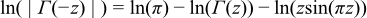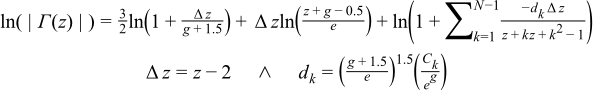#Boost C++ Libraries

...one of the most highly regarded and expertly designed C++ library projects in the world.

This is the documentation for an old version of boost. Click here for the latest Boost documentation.

#### Log Gamma

##### Synopsis

```#include <boost/math/special_functions/gamma.hpp>
```

```namespace boost{ namespace math{

template <class T>
calculated-result-type lgamma(T z);

template <class T, class Policy>
calculated-result-type lgamma(T z, const Policy&);

template <class T>
calculated-result-type lgamma(T z, int* sign);

template <class T, class Policy>
calculated-result-type lgamma(T z, int* sign, const Policy&);

}} // namespaces
```
##### Description

The lgamma function is defined by:The second form of the function takes a pointer to an integer, which if non-null is set on output to the sign of tgamma(z).

The final Policy argument is optional and can be used to control the behaviour of the function: how it handles errors, what level of precision to use etc. Refer to the policy documentation for more details.There are effectively two versions of this function internally: a fully generic version that is slow, but reasonably accurate, and a much more efficient approximation that is used where the number of digits in the significand of T correspond to a certain Lanczos approximation. In practice, any built-in floating-point type you will encounter has an appropriate Lanczos approximation defined for it. It is also possible, given enough machine time, to generate further Lanczos approximation's using the program libs/math/tools/lanczos_generator.cpp.

The return type of these functions is computed using the result type calculation rules: the result is of type `double` if T is an integer type, or type T otherwise.

##### Accuracy

The following table shows the peak errors (in units of epsilon) found on various platforms with various floating point types, along with comparisons to the GSL-1.9, GNU C Lib, HP-UX C Library and Cephes libraries. Unless otherwise specified any floating point type that is narrower than the one shown will have effectively zero error.

Note that while the relative errors near the positive roots of lgamma are very low, the lgamma function has an infinite number of irrational roots for negative arguments: very close to these negative roots only a low absolute error can be guaranteed.

Significand Size

Platform and Compiler

Factorials and Half factorials

Values Near Zero

Values Near 1 or 2

Values Near a Negative Pole

53

Win32 Visual C++ 8

Peak=0.88 Mean=0.14

(GSL=33) (Cephes=1.5)

Peak=0.96 Mean=0.46

(GSL=5.2) (Cephes=1.1)

Peak=0.86 Mean=0.46

(GSL=1168) (Cephes~500000)

Peak=4.2 Mean=1.3

(GSL=25) (Cephes=1.6)

64

Linux IA32 / GCC

Peak=1.9 Mean=0.43

(GNU C Lib Peak=1.7 Mean=0.49)

Peak=1.4 Mean=0.57

(GNU C Lib Peak= 0.96 Mean=0.54)

Peak=0.86 Mean=0.35

(GNU C Lib Peak=0.74 Mean=0.26)

Peak=6.0 Mean=1.8

(GNU C Lib Peak=3.0 Mean=0.86)

64

Linux IA64 / GCC

Peak=0.99 Mean=0.12

(GNU C Lib Peak 0)

Pek=1.2 Mean=0.6

(GNU C Lib Peak 0)

Peak=0.86 Mean=0.16

(GNU C Lib Peak 0)

Peak=2.3 Mean=0.69

(GNU C Lib Peak 0)

113

HPUX IA64, aCC A.06.06

Peak=0.96 Mean=0.13

Peak=0.99 Mean=0.53

Peak=0.9 Mean=0.4

Peak=3.0 Mean=0.9

##### Testing

The main tests for this function involve comparisons against the logs of the factorials which can be independently calculated to very high accuracy.

Random tests in key problem areas are also used.

##### Implementation

The generic version of this function is implemented by combining the series and continued fraction representations for the incomplete gamma function:where l is an arbitrary integration limit: choosing `l = max(10, a)` seems to work fairly well. For negative z the logarithm version of the reflection formula is used:For types of known precision, the Lanczos approximation is used, a traits class `boost::math::lanczos::lanczos_traits` maps type T to an appropriate approximation. The logarithmic version of the Lanczos approximation is:Where Le,g is the Lanczos sum, scaled by eg.

As before the reflection formula is used for z < 0.

When z is very near 1 or 2, then the logarithmic version of the Lanczos approximation suffers very badly from cancellation error: indeed for values sufficiently close to 1 or 2, arbitrarily large relative errors can be obtained (even though the absolute error is tiny).

For types with up to 113 bits of precision (up to and including 128-bit long doubles), root-preserving rational approximations devised by JM are used over the intervals [1,2] and [2,3]. Over the interval [2,3] the approximation form used is:

```lgamma(z) = (z-2)(z+1)(Y + R(z-2));
```

Where Y is a constant, and R(z-2) is the rational approximation: optimised so that it's absolute error is tiny compared to Y. In addition small values of z greater than 3 can handled by argument reduction using the recurrence relation:

```lgamma(z+1) = log(z) + lgamma(z);
```

Over the interval [1,2] two approximations have to be used, one for small z uses:

```lgamma(z) = (z-1)(z-2)(Y + R(z-1));
```

Once again Y is a constant, and R(z-1) is optimised for low absolute error compared to Y. For z > 1.5 the above form wouldn't converge to a minimax solution but this similar form does:

```lgamma(z) = (2-z)(1-z)(Y + R(2-z));
```

Finally for z < 1 the recurrence relation can be used to move to z > 1:

```lgamma(z) = lgamma(z+1) - log(z);
```

Note that while this involves a subtraction, it appears not to suffer from cancellation error: as z decreases from 1 the `-log(z)` term grows positive much more rapidly than the `lgamma(z+1)` term becomes negative. So in this specific case, significant digits are preserved, rather than cancelled.

For other types which do have a Lanczos approximation defined for them the current solution is as follows: imagine we balance the two terms in the Lanczos approximation by dividing the power term by its value at z = 1, and then multiplying the Lanczos coefficients by the same value. Now each term will take the value 1 at z = 1 and we can rearrange the power terms in terms of log1p. Likewise if we subtract 1 from the Lanczos sum part (algebraically, by subtracting the value of each term at z = 1), we obtain a new summation that can be also be fed into log1p. Crucially, all of the terms tend to zero, as z -> 1:The Ck terms in the above are the same as in the Lanczos approximation.

A similar rearrangement can be performed at z = 2:Copyright © 2006 , 2007, 2008 John Maddock, Paul A. Bristow, Hubert Holin, Xiaogang Zhang and Bruno Lalande Distributed under the Boost Software License, Version 1.0. (See accompanying file LICENSE_1_0.txt or copy at http://www.boost.org/LICENSE_1_0.txt)